# Distance Speed Time Formula

Distance Speed Time Formula

Speed is a measure of how quickly an object moves from one place to another. It is equal to the distance traveled divided by the time. It is possible to find any of these three values using the other two. This picture is helpful:The positions of the words in the triangle show where they need to go in the equations. To find the speed, distance is over time in the triangle, so speed is distance divided by time. To find distance, speed is beside time, so distance is speed multiplied by time.,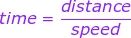,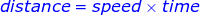,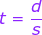,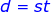s = speed (meters/second)

d = distance traveled (meters)

t = time (seconds)

Distance Speed Time Formula Questions:

1) A dog runs from one side of a park to the other. The park is 80.0 meters across. The dog takes 16.0 seconds to cross the park. What is the speed of the dog?

Answer: The distance the dog travels and the time it takes are given. The dog’s speed can be found with the formula: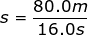s = 5.0 m/s

The speed of the dog is 5.0 meters per second.

2) A golf cart is driven at its top speed of 27.0 km/h for 10.0 minutes. In meters, how far did the golf cart travel?

Answer: The first step to solve this problem is to change the units of the speed and time so that the answer found will be in meters, since this is what the question asks for. The speed is:

s = 27.0 km/hs = 7.50 m/s

Converting the units, the speed is 7.50 m/s. The time the cart traveled for was:

t = 10.0 mint = 600s

The speed of the cart and the time of travel are given, so the distance traveled can be found using the formula:

d = st

d = (7.50 m/s)(600 s)

d = 4500 m

The golf cart traveled 4500 m, which is equal to 4.50 km.

 Related Links: Speed Quiz Speed and Velocity Quiz Angular Speed Formula Average Speed Formula